# Solve Math Problems In Edge Browser With Math Solver

Microsoft Math Solver is an interactive math learning tool for students that can quickly solve math problems. It can scan images of math problems and also offers a keyboard to type in different math problems.

This interactive tool is available in Microsoft Edge version 91 as a preview feature.

Here is how to enable and use it:

First, check the version of Edge browser by typing in the address bar:

edge://settings/help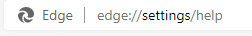If the version is 91, then Math Solver will be available.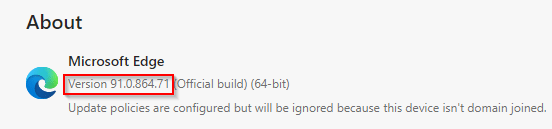To enable it next to the Edge address bar, change the appearance settings by typing in the address bar:

edge://settings/appearance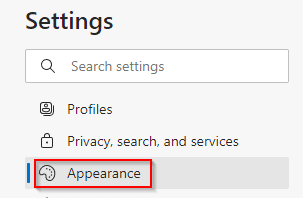From the Appearance section, enable the slider for Show Math Solver button.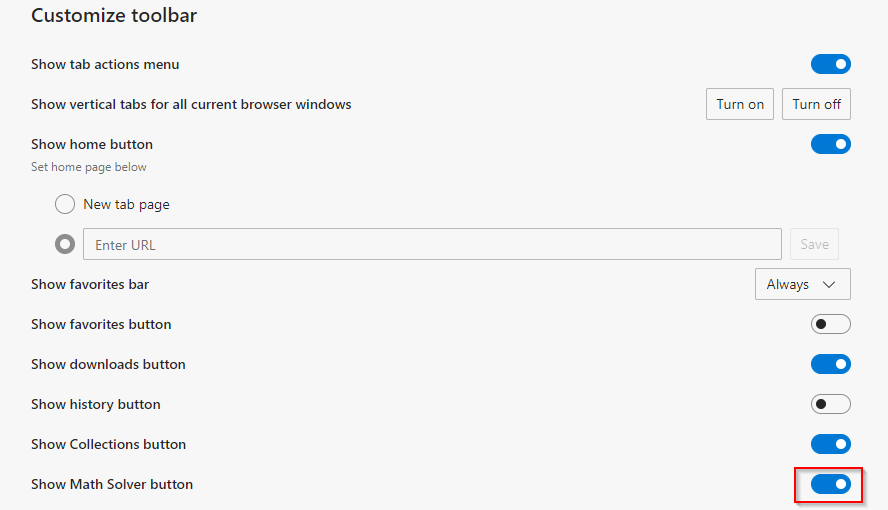It will now be available as an icon next to the address bar for quick access.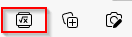Alternatively, click on the dots icon in Edge and choose More tools > Math Solver.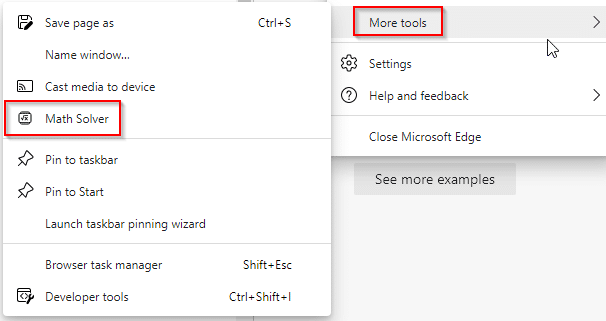However, having the icon enabled next to the address bar is easier and quicker for accessing it.

Once opened, it will be available in the sidebar.

## Using Math Solver: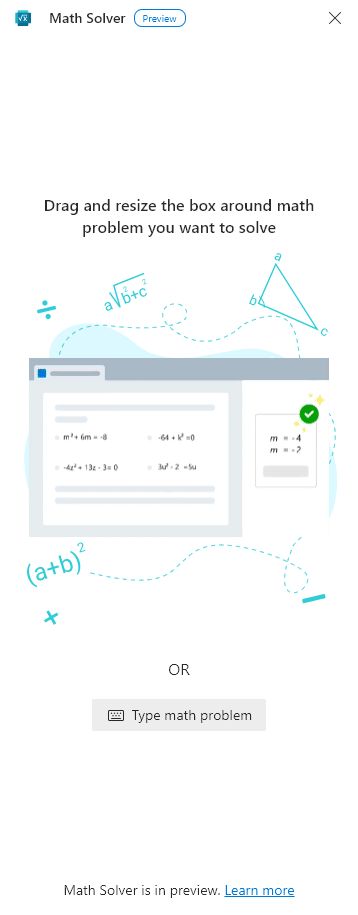It has two options: selecting the math problem using the drag and resize box or typing in the math problem.

### Selecting the math problem:

For this, you can take a photo of the math problem printed or handwritten as Math Solver recognizes both. Open the math problem image in Edge and then drag and resize the box over it.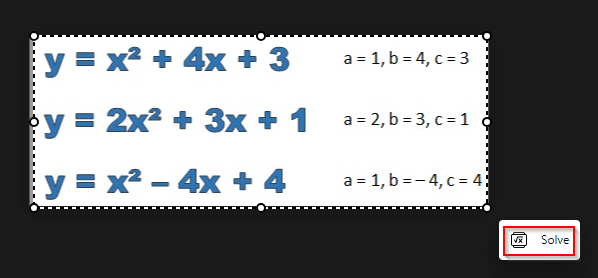[ Image courtesy: Mathematics Monster ]

There will be a small pop-up at the bottom of the box labeled Solve. Click on it.

Math Solver will automatically detect and process the math problem.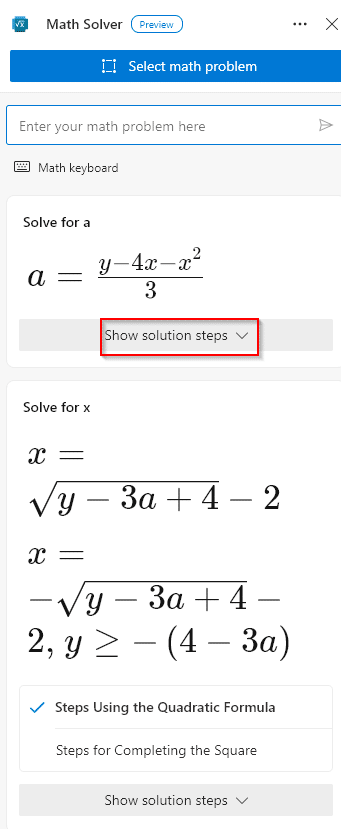The solution for it will be available in the sidebar. Also, clicking on the Show solution steps menu will explain each of these steps involved in solving the problem.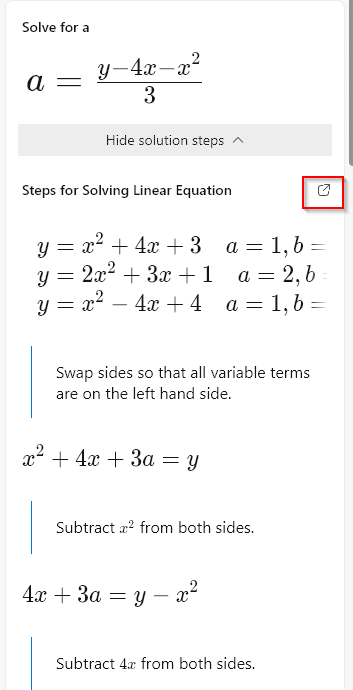At the end of the solution are useful, related resources that help understand similar math problems with the related concepts.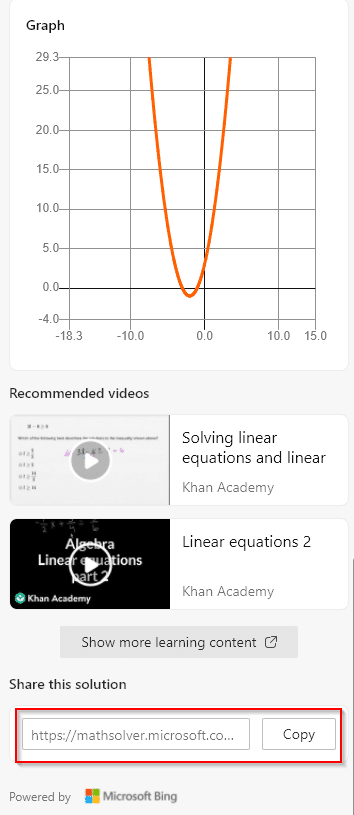The solution link can also be shared with others if needed.

Also, you can open the solution in a new tab by clicking on the pop-out icon. It will open Math Solver with a detailed view that has different math topics and related examples listed.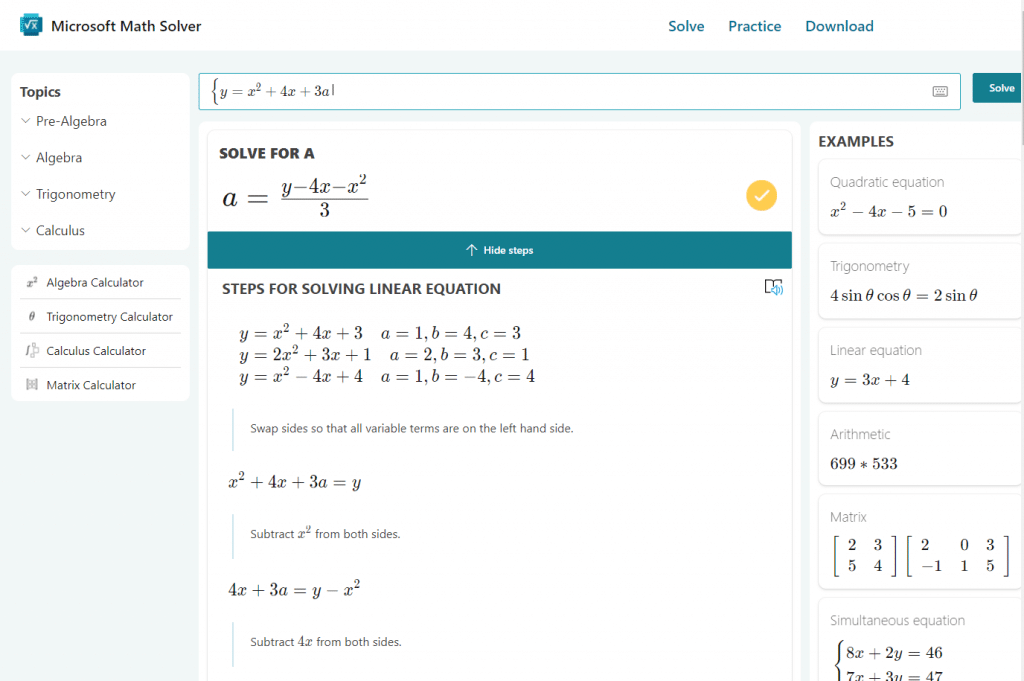Pretty cool.

### Typing the math problem:

Besides the drag and resize option, you can also directly type in the problem. Click on the Math Solver icon and choose Type math problem.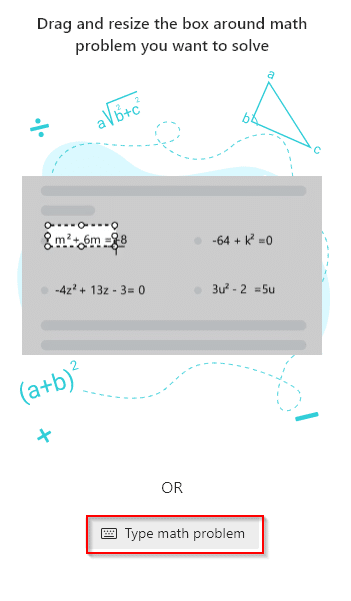A math keyboard will be visible. It will have different functions in a row, and you can choose the required ones from there.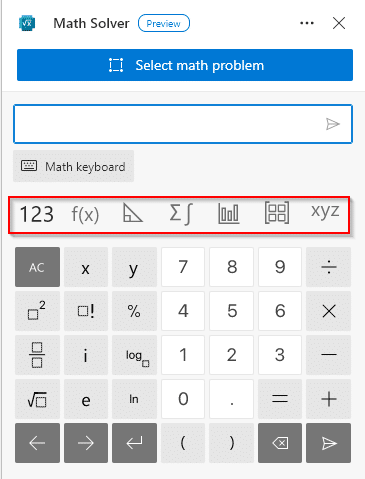For example, clicking on the calculus button from the row will open the inputs for typing in calculus problems.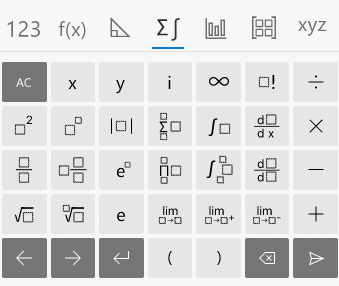Similarly, the trigonometry option will open the inputs for those problems, and so on.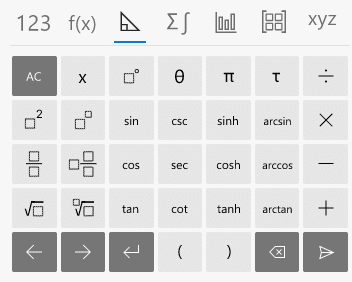After typing in the problem, click on the go icon next to it.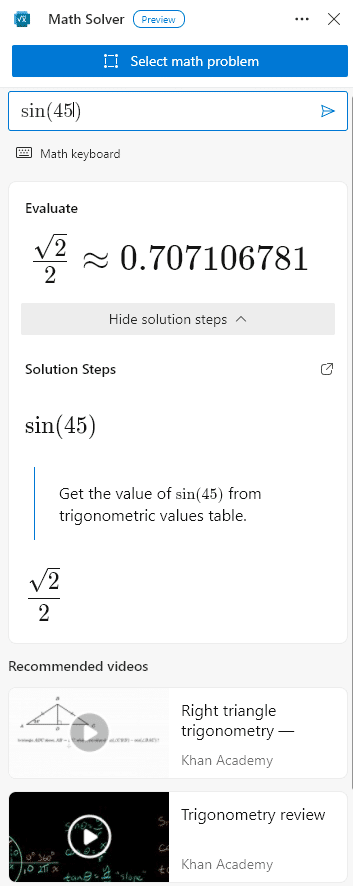Math Solver will process and list the solution for these problems.

It is a useful tool that makes learning math for students simple and interactive.

Hopefully, it will be a permanent addition to the subsequent Edge versions as it is in preview mode for now.

Do try it out.